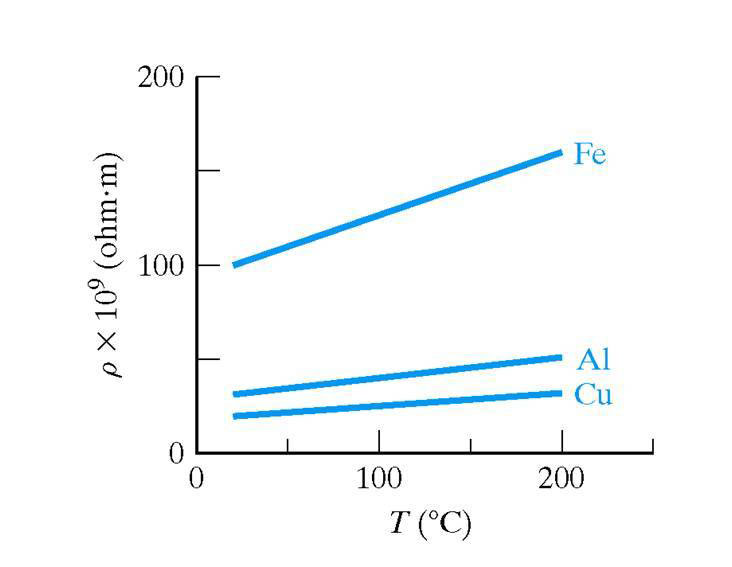# Temperature effect on conductivity

An intrinsic semiconductor at absolute zero, behaves as a perfect insulator. The amount of energy required to break a covalent bond is 0.72 eV in case of germanium and 1.11 eV in case of silicon. Equivalently, we say that the energy needed for lifting an electron from the valence band to the conduction band is 0.72 eV for germanium and 1.11 eV for silicon. When an electron jumps the forbidden energy gap, it leaves a hole in the valence band. Note that the value of forbidden energy gap (EG) is more in case of silicon than that of germanium. Therfore, less number of covalent bonds will be broken and less number of electron hole pairs will be generated in silicon than in germanium at room temperature. At room temperature the conductivity of silicon will be less than that of germanium.At room temperature, because of thermal energy, some of elctron hole pairs are generated. For example, in a sample of germanium at room temperature, the intrinsic carrier concentration is 2.5 x 1019 per m3. The intrinsic semiconductor has a very small conductivity. Now, if we rise the temperature further, more electrons hole pairs are generated. The higher the temperature, the higher is the concentration of charge carriers. As more charge carriers are made available. The conductivity of intrinsic semiconductor increases with in increase in temperature. In other words, the resistivity of an intrinsic semiconductors decreases as the temperature increases. That is the intrinsic semiconductor have negative temperature coefficient of resistance.